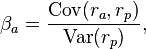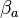222

# Why Accounting Education Important# Why Accounting Education Volunteer## Become Volunteer Teacher

In finance, beta or beta coefficient is important term for checking the changing in the value or return of any financial asset by creating its relation with the market value or return of same financial asset.

There three parts of beta coefficient

1. Beta Zero

If there is not any effect of market return changes on any financial asset's return changes, it will be beta zero. For example, govt. treasury bond has no risk of market changes, these provide us same return.

2. Positive Beta

If any financial asset's return increases or decreases due to increasing or decreasing the market return, then this will the positive beta.

3. Negative Beta

If any financial asset's return decreases due to increasing the market return or opposite relation between the both return, then it will be the negative beta.

Following is formula of betais called the asset's beta coefficient. ra measures the rate of return of the asset, rp measures the rate of return of the portfolio, and cov(ra,rp) is the covariance between the rates of return.

For calculating of beta, we have to learn linear regression and regression analysis (line of best fit) which you can find in statistics book. If you are investor, beta can be helpful for you. If your stock or financial asset has beta 2 or higher value, there is more possibility of growing your stock's return by growing the market overall return. Because beta is just co-relation of your return with market return.

Example of Company's beta

We have just search Google Inc. in google finance and its beta is 1.09 which is showing right side just above its graph, following is its screen cast.

Simple Calculation of Beta

through Capital Asset Pricing Model, we can calculate beta:

Required Rate of Return = Risk free rate of return + ( Beta X Market premium rate of return )

If risk free rate is 3% or 0.03, market premium rate is 6% or 0.06 and required rate of return is 14% or 0.14, then beta is

0.14 = 0.03 + Beta X 0.06

0.14 - 0.03 = Beta X 0.06

0.11 / 0.06 = Beta

1.83 = Beta

Related : CAPMName

ltr
item
Accounting Education: Beta Coefficient in Finance
Beta Coefficient in Finance